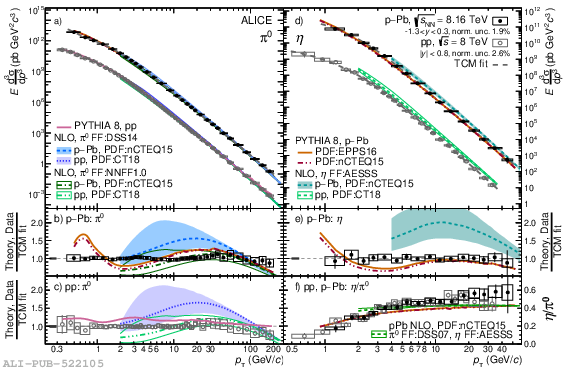# Figure 3

 Neutral pion a) and $\eta$ meson d) \com{invariant differential} cross sections for \pp\ collisions at $\sUp$ and $\pPb$ collisions at $\sU$ together with TCM fits, NLO calculations  and PYTHIA 8  predictions using different (n)PDFs . Statistical uncertainties are shown as vertical bars; the systematic uncertainties as boxes. The ratios of the $\pz$ spectra in \pPb\ and \pp\ collisions to the TCM fits are shown in panel b) and c), respectively, together with the ratios of the calculations to the fits; panel e) shows the same for $\eta$ mesons in \pPb\ collisions. In panel f) the $\eta/\pi^0$ ratios in \pp\ and \pPb\ collisions are compared to theory predictions The normalization uncertainty in the spectra ratio panels is indicated as a solid gray box around unity.